# Introduction to Neural Networks

~ by @raphaelmcobe ~

## Neural Networks

• Neurons as structural constituents of the brain [Ramón y Cajál, 1911];
• Five to six orders of magnitude slower than silicon logic gates;
• In a silicon chip happen in the nanosecond (on chip) vs millisecond range (neural events);
• A truly staggering number of neurons (nerve cells) with massive interconnections between them;

## Neural Networks

• Receive input from other units and decides whether or not to fire;
• Approximately 10 billion neurons in the human cortex, and 60 trillion synapses or connections [Shepherd and Koch, 1990];
• Energy efficiency of the brain is approximately $10^{−16}$ joules per operation per second against ~ $10^{−8}$ in a computer;

## Neurons

• input signals from its dendrites;
• output signals along its (single) axon;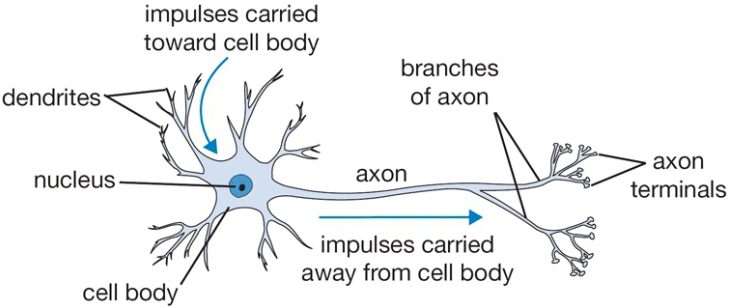## Neurons

### How do they work?

• Control the influence from one neuron on another:
• Excitatory when weight is positive; or
• Inhibitory when weight is negative;
• Nucleus is responsible for summing the incoming signals;
• If the sum is above some threshold, then fire!

## Neurons

### Artificial Neuron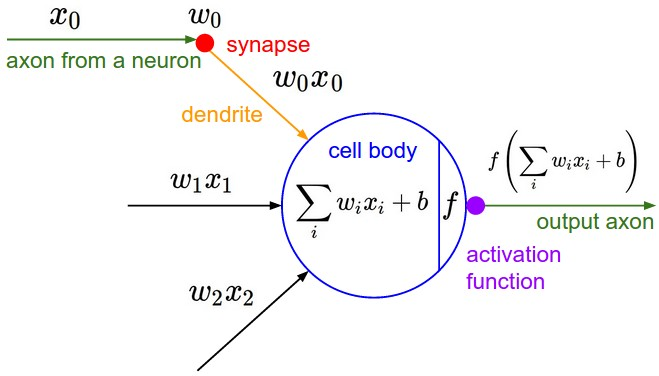## Neural Networks

• It appears that one reason why the human brain is so powerful is the sheer complexity of connections between neurons;
• The brain exhibits huge degree of parallelism;

## Artificial Neural Networks

• Model each part of the neuron and interactions;
• Interact multiplicatively (e.g. $w_0x_0$) with the dendrites of the other neuron based on the synaptic strength at that synapse (e.g. $w_0$ );
• Learn synapses strengths;

## Artificial Neural Networks

### Function Approximation Machines

• Datasets as composite functions: $y=f^{*}(x)$
• Maps $x$ input to a category (or a value) $y$;
• Learn synapses weights and aproximate $y$ with $\hat{y}$:
• $\hat{y} = f(x;w)$
• Learn the $w$ parameters;

## Artificial Neural Networks

• Can be seen as a directed graph with units (or neurons) situated at the vertices;
• Some are input units
• Receive signal from the outside world;
• The remaining are named computation units;
• Each unit produces an output
• Transmitted to other units along the arcs of the directed graph;

## Artificial Neural Networks

• Input, Output, and Hidden layers;
• Hidden as in “not defined by the output”;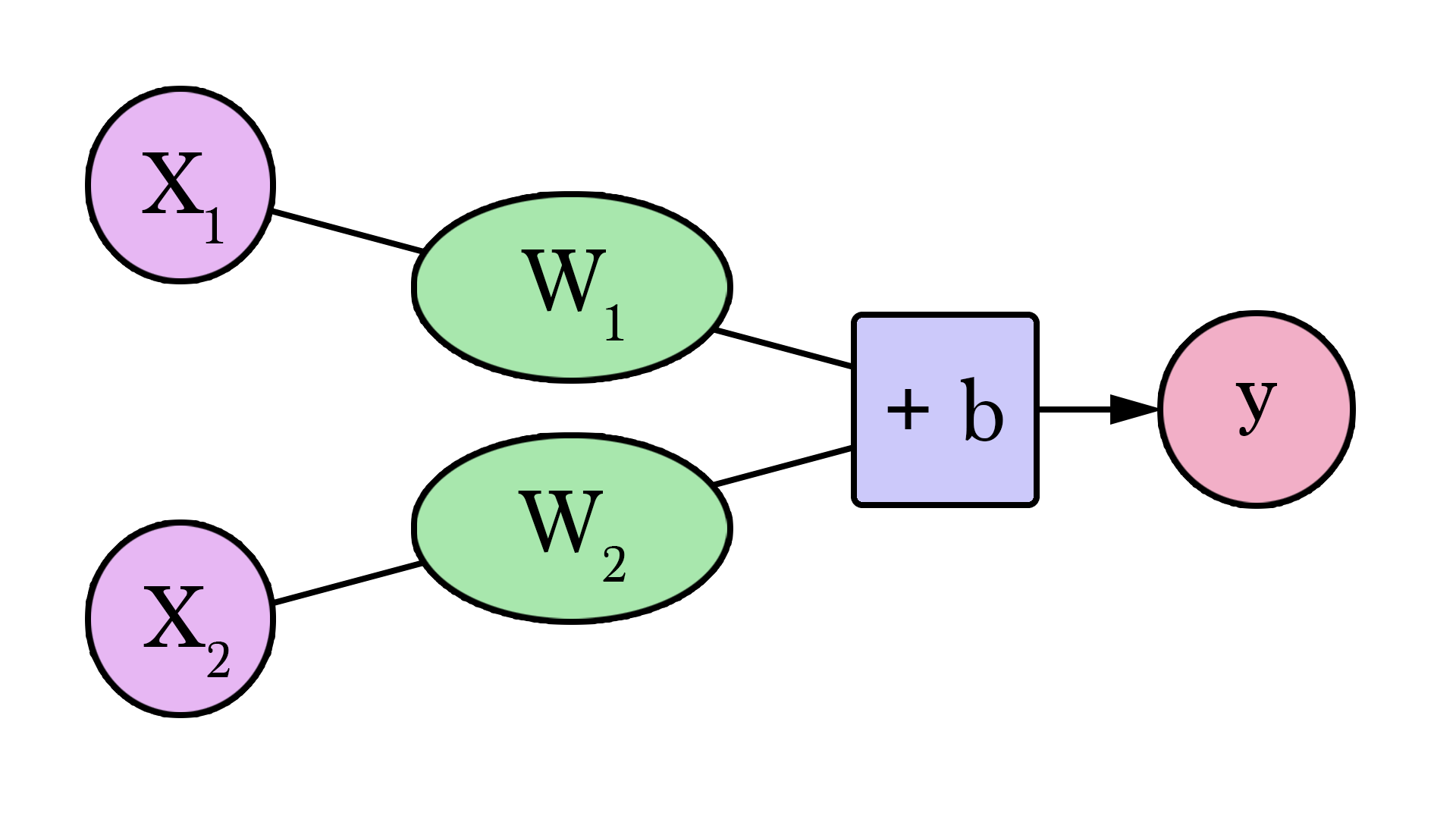## Artificial Neural Networks

###### Motivation Example (taken from Jay Alammar blog post)
• Imagine that you want to forecast the price of houses at your neighborhood;
• After some research you found that 3 people sold houses for the following values:

Area (sq ft) (x) Price (y)
2,104 $\$399,900$1,600$\$329,900$

## Artificial Neural Networks

###### Motivation Example (taken from Jay Alammar blog post)
• Our very first neural network looks like this: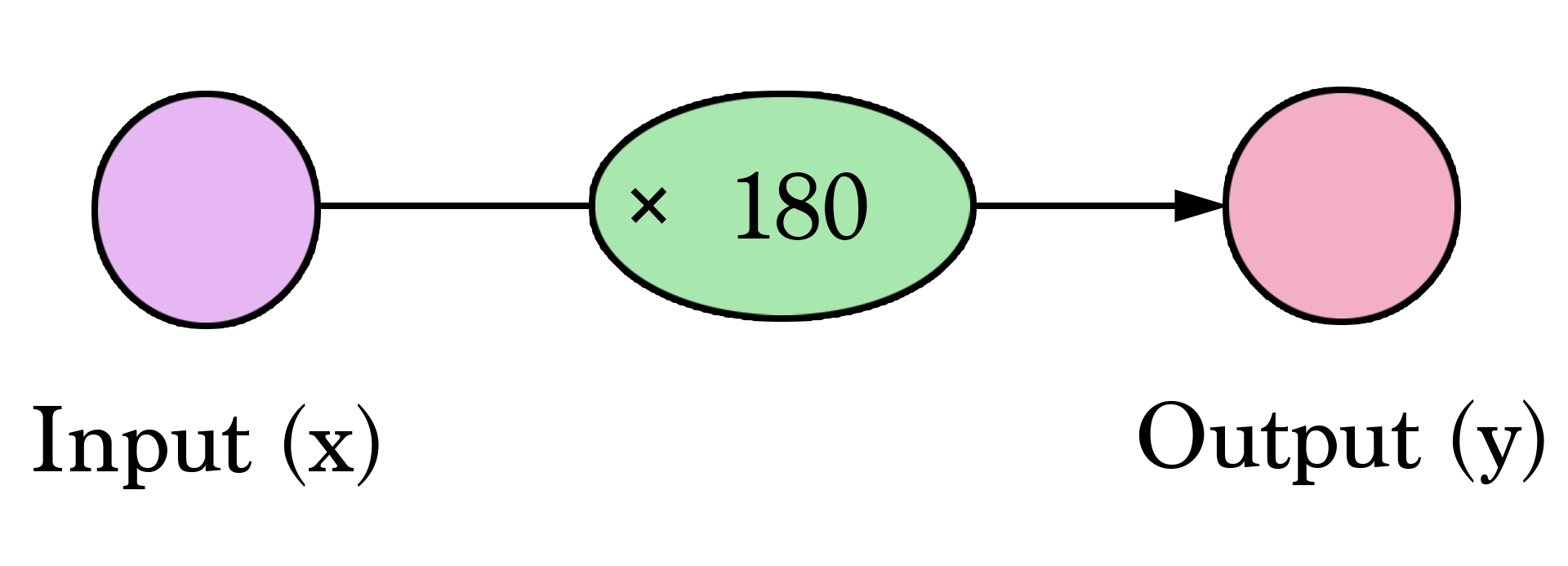## Artificial Neural Networks

• Multiplying $2,000$ sq ft by $180$ gives us $\$360,000$. • Calculating the prediction is simple multiplication. • We needed to think about the weight we’ll be multiplying by. • That is what training means! Area (sq ft) (x) Price (y) Estimated Price($\hat{y}$) 2,104$\$399,900$ $\$378,720$1,600$\$329,900$ $\$288,000$2,400$\$369,000$ $\$432,000$## Artificial Neural Networks ###### Motivation Example (taken from Jay Alammar blog post) • How bad is our model? • Calculate the Error; • A better model is one that has less error; Mean Square Error :$2,058$Area (sq ft) (x) Price (y) Estimated Price($\hat{y}$)$y-\hat{y}(y-\hat{y})^2$2,104$\$399,900$ $\$378,720\$21$ $449$
1,600 $\$329,900\$288,000$ $\$421756$2,400$\$369,000$ $\$432,000\$-63$ $3969$

## Artificial Neural Networks

• Fitting the line to our data: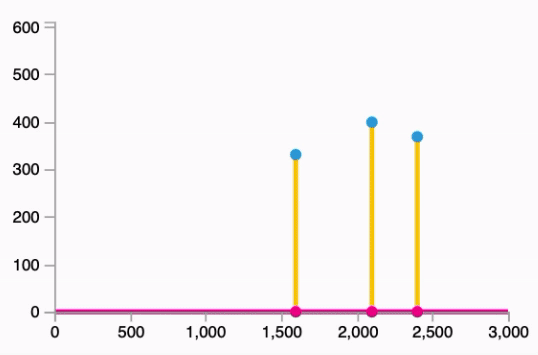Follows the equation: $\hat{y} = W * x$

## Artificial Neural Networks

$\hat{y}=Wx + b$

## Artificial Neural Networks

### The Bias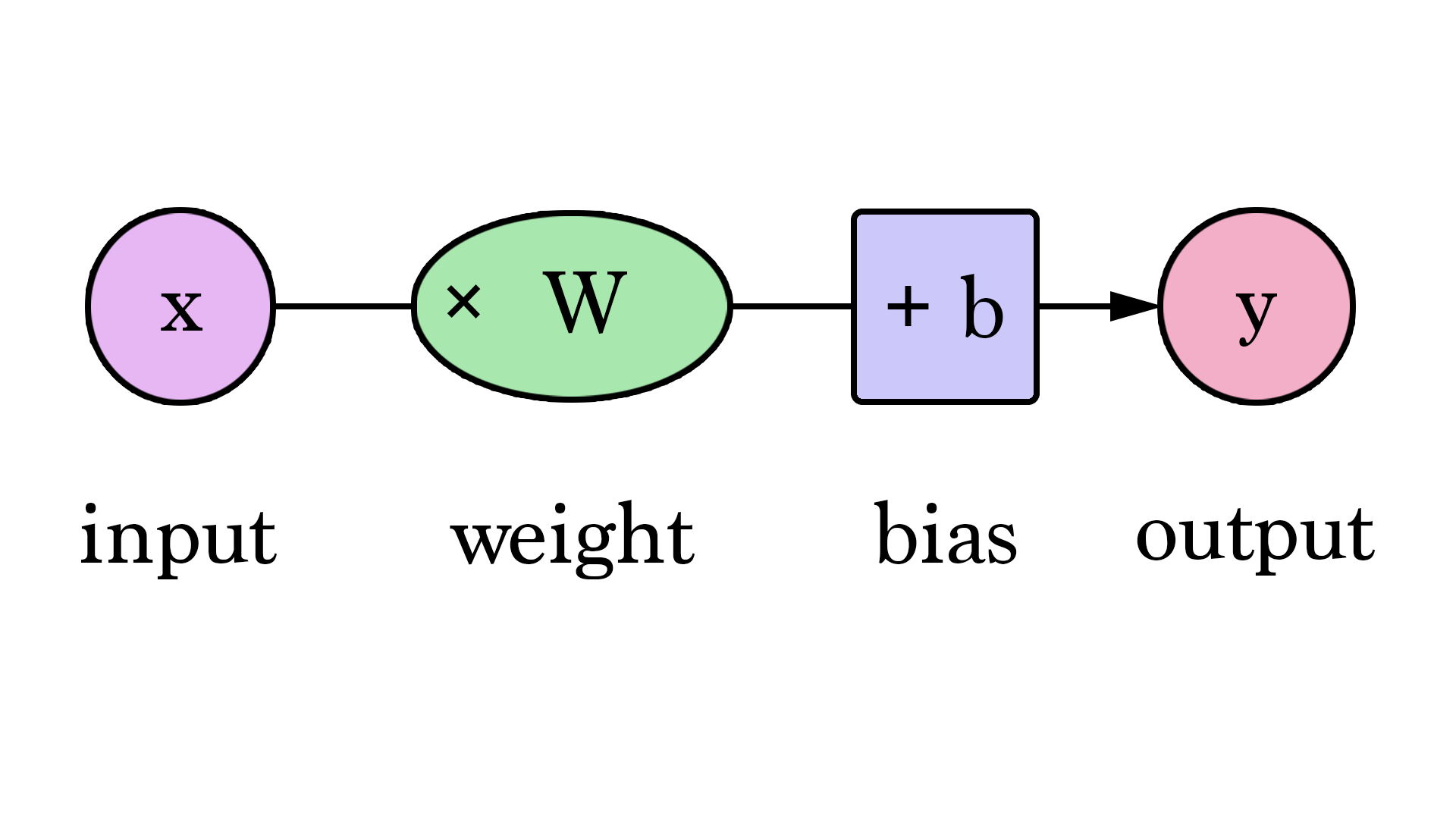## Artificial Neural Networks

### How to discover the correct weights?

• Finding the minimum of a function;
• Look for the best weights values, minimizing the error;
• Takes steps proportional to the negative of the gradient of the function at the current point.
• Gradient is a vector that is tangent of a function and points in the direction of greatest increase of this function.

## Artificial Neural Networks

• In mathematics, gradient is defined as partial derivative for every input variable of function;
• Negative gradient is a vector pointing at the greatest decrease of a function;
• Minimize a function by iteratively moving a little bit in the direction of negative gradient;

## Artificial Neural Networks

• With a single weight: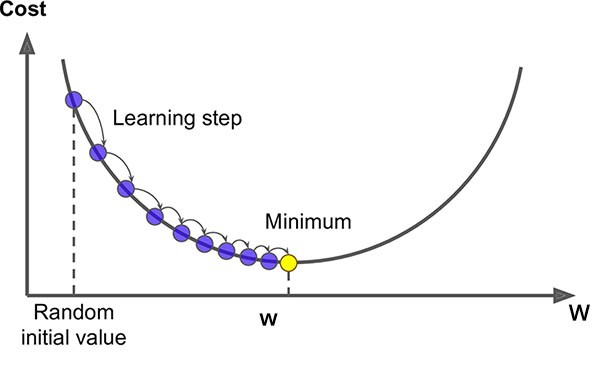## Artificial Neural Networks

### Perceptron

• In 1958, Frank Rosenblatt proposed an algorithm for training the perceptron.
• Simplest form of Neural Network;
• One unique neuron;

## Artificial Neural Networks

### Perceptron

• Classification of observations into two classes: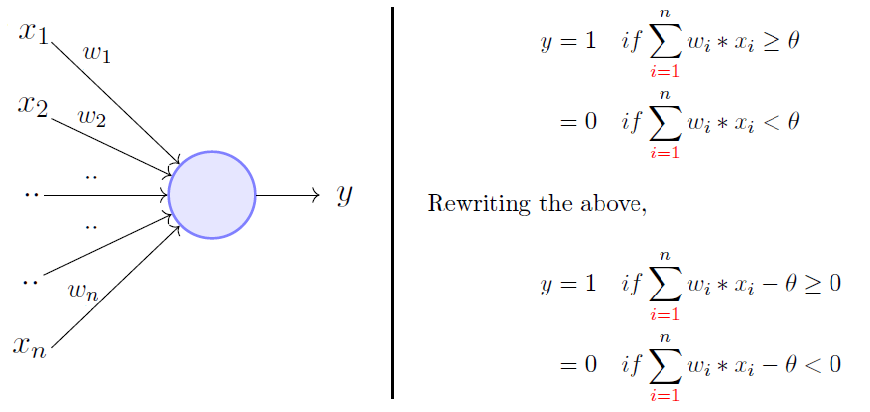## Artificial Neural Networks

### Perceptron

• Classification of observations into two classes: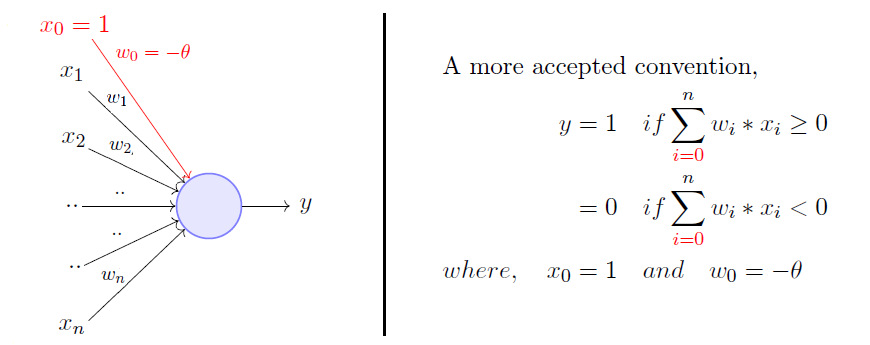## Artificial Neural Networks

### Perceptron

• E.g, the OR function: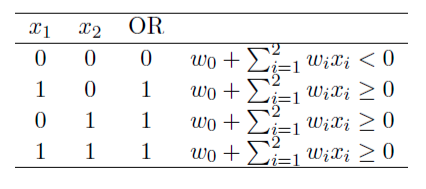## Artificial Neural Networks

### Perceptron

• E.g, the OR function: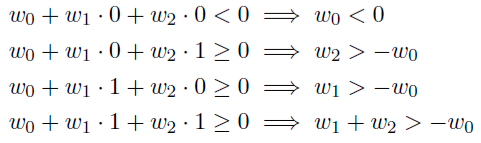## Artificial Neural Networks

### Perceptron

• One possible solution $w_0=-1$, $w_1=1.1$, $w_2=1.1$: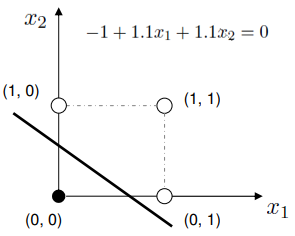## Artificial Neural Networks

### The Keras framework

• High-level neural networks API;
• Capable of running on top of TensorFlow, CNTK, or Theano;
• Focus on enabling fast experimentation;
• Go from idea to result with the least possible delay;
• Runs seamlessly on CPU and GPU;
• Compatible with: Python 2.7-3.6;

## Artificial Neural Networks

### The Keras framework

• Use the implementation of the tensorflow:

• Create a sequential model (perceptron)

# Import the Sequential model
from tensorflow.keras.models import Sequential

# Instantiate the model
model = Sequential()


## Artificial Neural Networks

### The Keras framework

• Create a single layer with a single neuron:

• units represent the number of neurons;

# Import the Dense layer
from tensorflow.keras.layers import Dense

# Add a forward layer to the model


## Artificial Neural Networks

### The Keras framework

• Compile and train the model

• The compilation creates a computational graph of the training;

# Specify the loss function (error) and the optimizer
#   (a variation of the gradient descent method)
model.compile(loss="mean_squared_error", optimizer="sgd")

# Fit the model using the train data and also
#   provide the expected result
model.fit(x=train_data_X, y=train_data_Y)


## Artificial Neural Networks

### The Keras framework

• Evaluate the quality of the model:

# Use evaluate function to get the loss and other metrics that the framework
#  makes available
loss_and_metrics = model.evaluate(train_data_X, train_data_Y)
print(loss_and_metrics)
#0.4043288230895996

# Do a prediction using the trained model
prediction = model.predict(train_data_X)
print(prediction)
# [[-0.25007164]
#  [ 0.24998784]
#  [ 0.24999022]
#  [ 0.7500497 ]]


## Artificial Neural Networks

### The Keras framework

#### Exercise:

Run the example of the Jupyter notebook:
Perceptron - OR

## Artificial Neural Networks

### Perceptron

#### Exercise:

• What about the AND function?
$x_1$ $x_2$ $y$
0 0 0
0 1 0
1 0 0
1 1 1

## Artificial Neural Networks

### Activation Functions

• Describes whether or not the neuron fires, i.e., if it forwards its value for the next neuron layer;
• Historically they translated the output of the neuron into either 1 (On/active) or 0 (Off) - Step Function:

if prediction[i]>0.5: return 1
return 0


## Artificial Neural Networks

### Activation Functions

• Multiply the input by its weights, add the bias and applies activation;
• Sigmoid, Hyperbolic Tangent, Rectified Linear Unit;
• Differentiable function instead of the step function;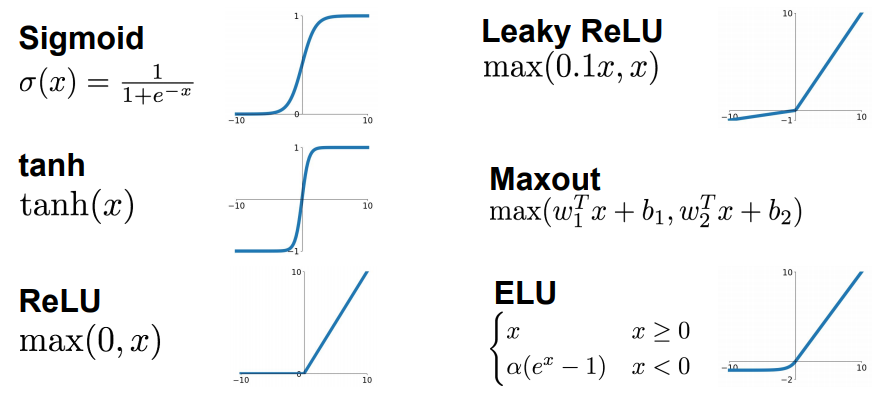## Artificial Neural Networks

### The Bias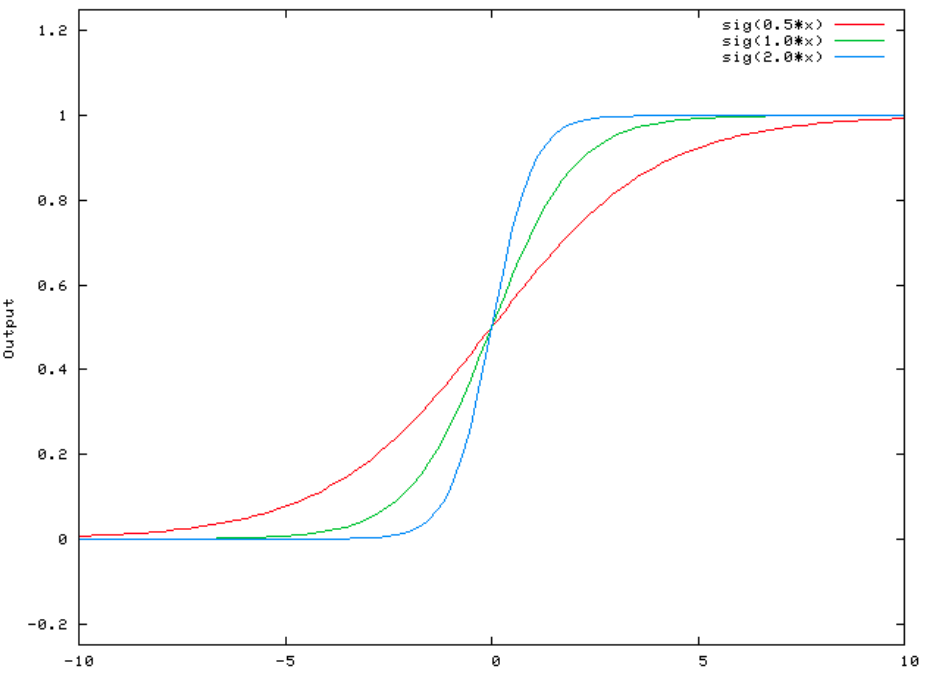## Artificial Neural Networks

### The Bias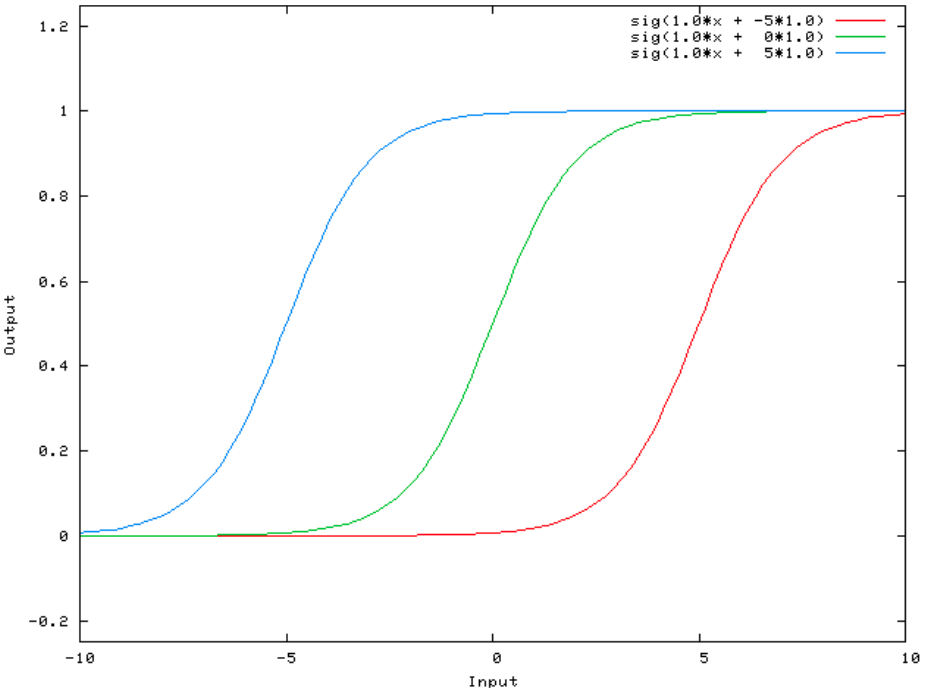## Artificial Neural Networks

### Perceptron - What it can’t do!

• The XOR function: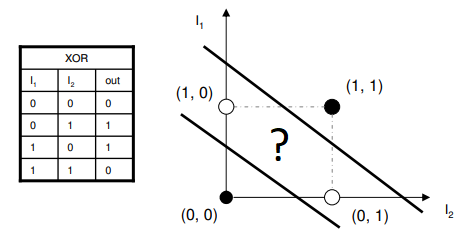## Artificial Neural Networks

### Perceptron - Solving the XOR problem

• 3D example of the solution of learning the OR function:
• Using Sigmoid function;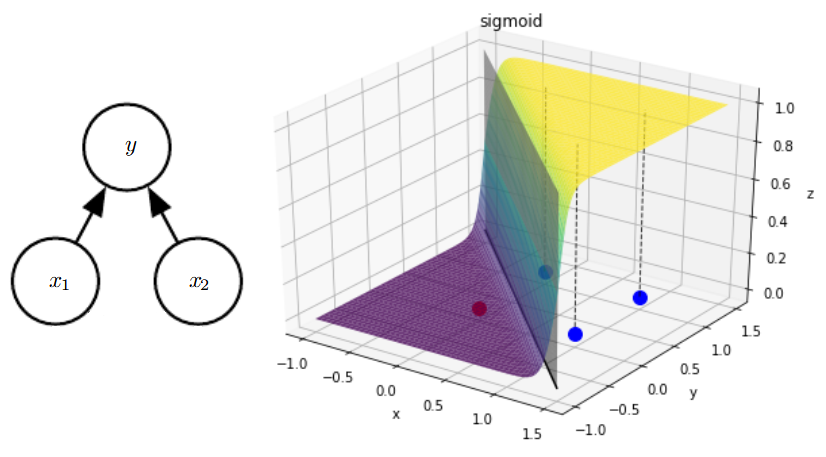## Artificial Neural Networks

### Perceptron - Solving the XOR problem

• Maybe there is a combination of functions that could create hyperplanes that separate the XOR classes:

## Artificial Neural Networks

### Perceptron - Solving the XOR problem

• Implementing an ANN that can solve the XOR problem:

• Add a new layer with a larger number of neurons:

...
#Create a layer with 4 neurons as output

# Connect to the first layer that we defined


## Artificial Neural Networks

#### Multilayer Perceptrons - Increasing the model power

• Typically represented by composing many different functions: $$y = f^{(3)}(f^{(2)}(f^{(1)}(x)))$$

• The depth of the network - the deep in deep learning! (-;

## Artificial Neural Networks

#### Multilayer Perceptrons - Increasing the model power

• Information flows from $x$ , through computations and finally to $y$.
• No feedback!

## Artificial Neural Networks

### Understanding the training

• Plot the architecture of the network:

tf.keras.utils.plot_model(model, show_shapes=True, show_layer_names=False)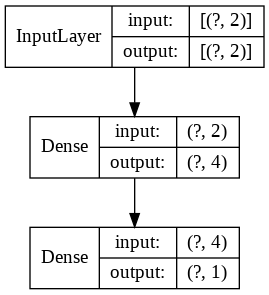## Artificial Neural Networks

### Understanding the training

• Plotting the training progress of the XOR ANN:

history = model.fit(x=X_data, y=Y_data, epochs=2500, verbose=0)
import matplotlib.pyplot as plt
plt.plot(history.history['loss'])
plt.title('Model Training Progression')
plt.ylabel('Loss')
plt.xlabel('Epoch')
plt.legend(['Loss'], loc='upper left')
plt.show()## Artificial Neural Networks

### Optimization alternatives

• The Gradient Descent is not always the best option to go with:
• Only does the update after calculating the derivative for the whole dataset;
• Can take a long time to find the minimum point;

## Artificial Neural Networks

### Optimization alternatives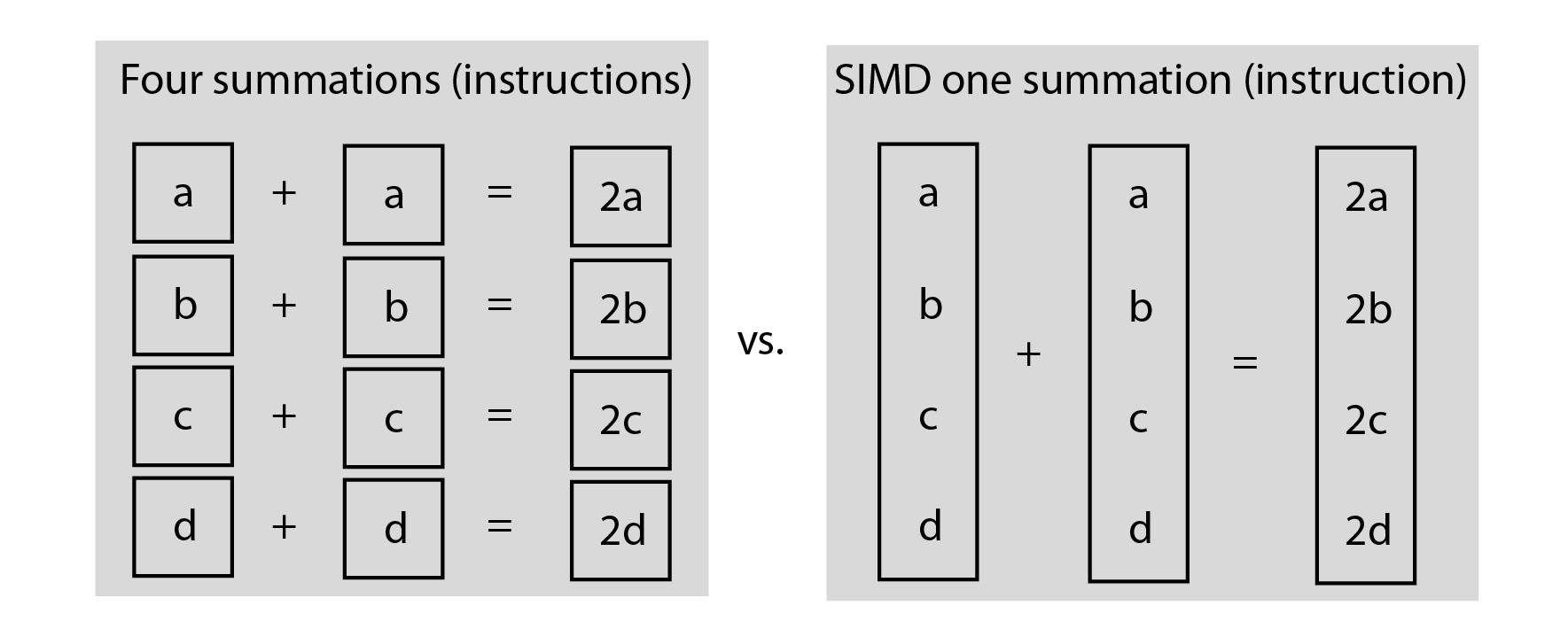## Artificial Neural Networks

### Multilayer Perceptron - XOR

• Try another optimizer:

model.compile(loss="mean_squared_error", optimizer="adam")

• My solution

## Artificial Neural Networks

### Predicting probabilities

• The Softmax function;
• Takes an array and outputs a probability distribution, i.e., the probability of the input example belonging to each of the classes in my problem;
• One of the activation functions available at Keras:

model.add(Dense(2, activation="softmax"))


## Artificial Neural Networks

### Loss functions

• For regression problems
• Mean squared error is not always the best one to go;
• What if we have a three classes problem?
• Alternatives: mean_absolute_error, mean_squared_logarithmic_error

## Artificial Neural Networks

### Loss functions

• Cross Entropy loss:
• Default loss function to use for binary classification problems.
• Measures the performance of a model whose output is a probability value between 0 and 1;
• Loss increases as the predicted probability diverges from the actual label;
• A perfect model would have a log loss of 0;

## Artificial Neural Networks

### Dealing with overfitting

• Dropout layers:
• Randomly disable some of the neurons during the training passes;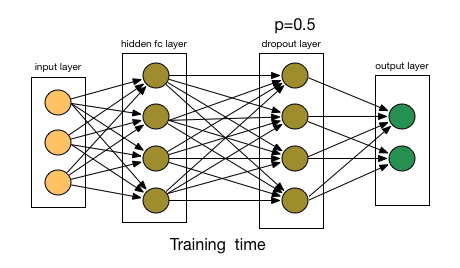## Artificial Neural Networks

### Dealing with overfitting

• Dropout layers:

# Drop half of the neurons outputs from the previous layer


## Artificial Neural Networks

### The MNIST MLP

• Try to improve the classification results using this notebook:
• Things to try:
• Increase the number of neurons at the first layer;
• Change the optimizer and the loss function;
• Try categorical_crossentropy and rmsprop optimizer;
• Try adding some extra layers;

## Artificial Neural Networks

### The MNIST MLP

• Try to improve the classification results using this notebook:
• Things to try:

• Try addind Dropout layers;
• Increase the number of epochs;
• Try to normalize the data!
• What is the best accuracy?

# The Exercise

## Artificial Neural Networks

### The Exercise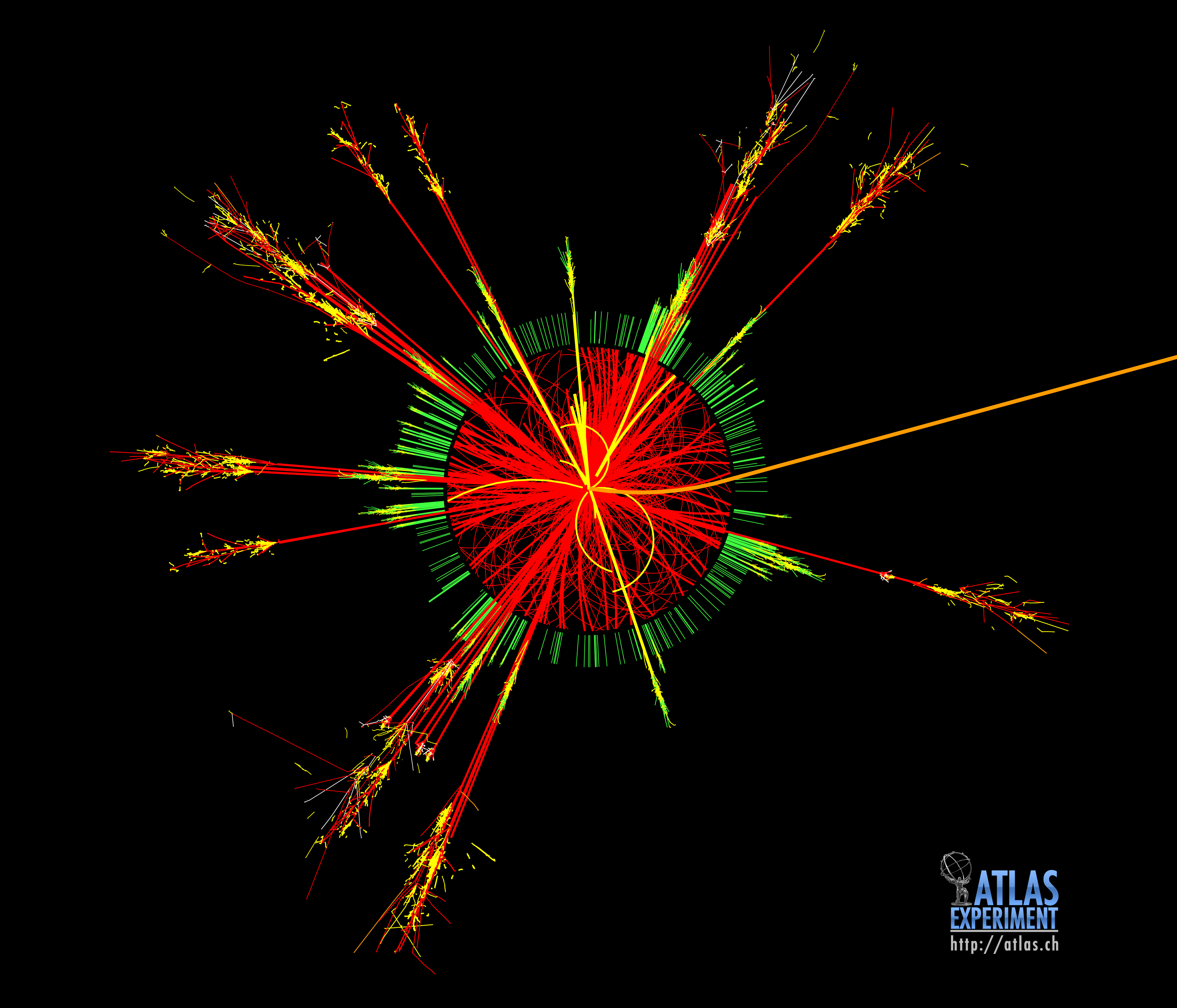## Artificial Neural Networks

### The Exercise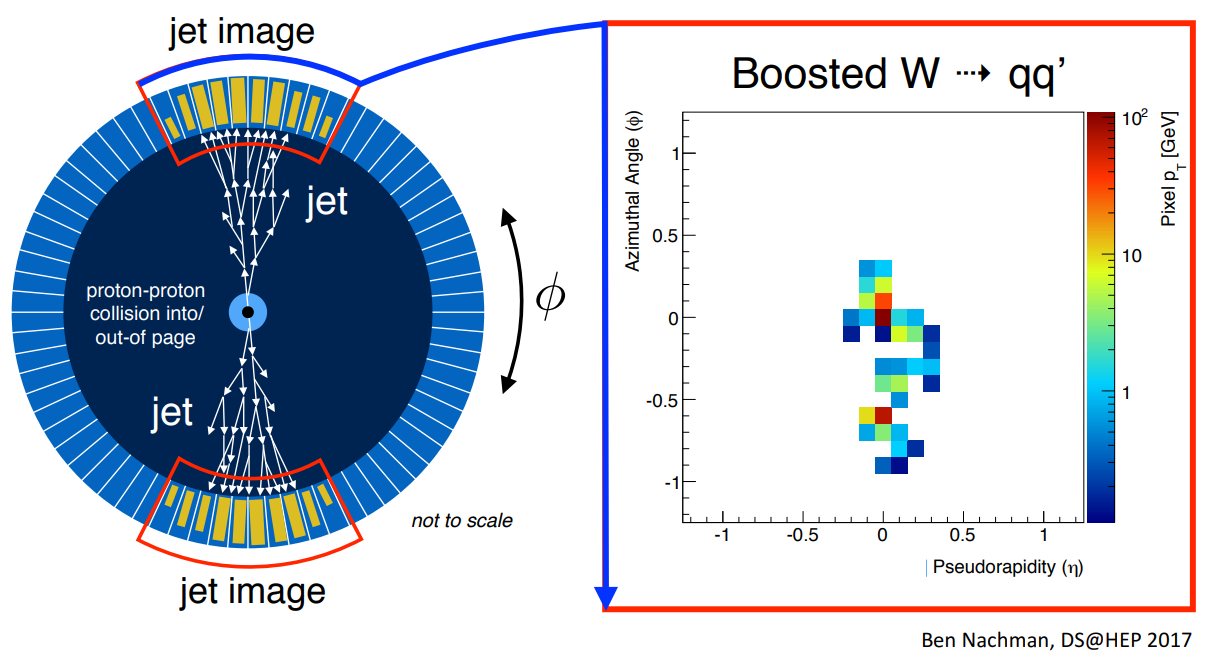## Artificial Neural Networks

### Signal VS Background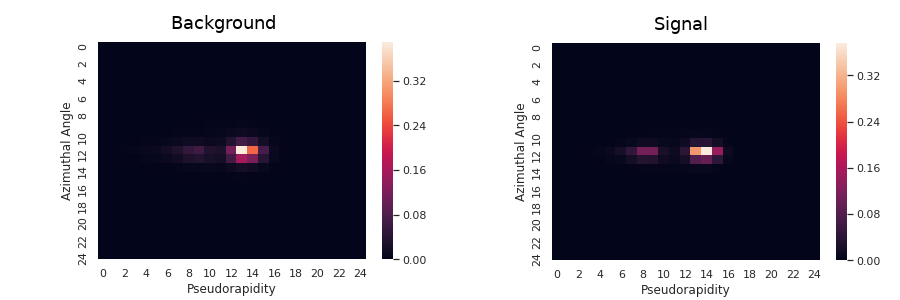## Artificial Neural Networks

### Signal VS Background

Run this Jupyter Notebook for performing the Jet Classification.